﻿ Theoretical Derivation of Ampere's Circuital LawPublications are Open
Access in this journal
Article Versions
Export Article
• Normal Style
• MLA Style
• APA Style
• Chicago Style
Original Article
Open Access Peer-reviewed

### Theoretical Derivation of Ampere's Circuital Law

Yiran Luo, Shimeng Feng
International Journal of Physics. 2020, 8(3), 105-107. DOI: 10.12691/ijp-8-3-3
Received July 14, 2020; Revised August 16, 2020; Accepted August 25, 2020

### Abstract

Amperes circuital law is a very important formula in classical electromagnetics. However, the corresponding detailed theoretical derivation is rarely seen in relevant textbooks. In this paper, based on the magnetic field produced by a moving charge, we taken the divergence theorem and rotation of the magnetic field, respectively, and given a series of mathematical transformations. At last, we derived Ampere's circuital law of the magnetic field, which is very helpful to understand the physical source of the law.

### 1. Introduction

Ampere circuital law is a very important formula in classical electromagnetics, but it is almost directly given in many textbooks. Using the space solid angle to calculate the circuital integral of the magnetic field produced by the current carrying wire, we can directly derive Ampere circuital law. In order to make Ampere circuital law applicable in the whole magnetic field, Maxwell extended it to the full current law, and it’s correctness was verified by all the conclusions obtained from Maxwell equations and the experimental facts.

For the magnetic field produced by the current carrying wire infinitely length, the theoretical derivation of Ampere's circuital law were reported. papers 1, 2, 3, 4 show that the law had been derived by quantum mechanics, geometry and pure mathematics, respectively. For example, using the quantum mechanical method 5, Through a series of approximate treatment, the law had been derived. however, it is difficult for ordinary students and physics teachers to understand the mathematical derivation process. So far, few papers have been reported that the theoretical derivation of Ampere's circuital law of magnetic field produced by a single moving charge or by the current carrying wire with finite length was been obtained. In this paper, based on the theoretical expression of the magnetic field generated by the moving charge, we taken the divergence and rotation of the magnetic field, respectively, and derived Ampere's law of the magnetic field, which is of much help to understand Ampere's law.

### 2. Theoretical Derivation

For a moving point charge (seen in Figure 1), the expression of its magnetic field intensity in space is as follows 6

• Figure 1. A charge moving uniformly in a straight line in free space(1)

here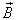is the magnetic induction intensity,is the dielectric constant in vacuum.is the speed of light in a vacuum.is the electric quantity of charge.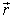Is the position vector from a point in space to the charge.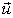is the velocity of moving charge. In this paper, the moving charge is in the same direction as the x-axis. Equation (1) can be also written as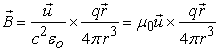(2)

Here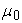is permeability in a vacuum. As we all know, the expression of electric displacement vector in vacuum is expressed as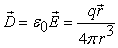(3)

here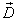is the electric displacement vector,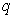is the electric quantity of charge,is the position vector from a point in space to the charge. Substituting equation (3) into equation (2), we get(4)

After two sides of equation(4) are multiplied by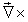at the same time, we have(5)

UsingEquation (5) can be changed into(6)

For a charge moving uniformly, the partial derivative ofat any direction is equal to zero, that is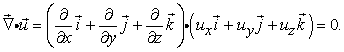(7)

Substitution of equation (7) into equation (6) produces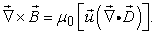(8)

For an infinite space with uniformly distributed charges (seen in Figure 2)

• Figure 2. An infinitely large space with uniformly distributed charges

According to Gauss's theorem of electric displacement flux in dielectric, the flux of electric displacement on the closed surface is equal to the amount of charge surrounded by the closed surface, that is(9)

here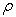is the volume density of charges, r is the radius of Gaussian sphere in Figure 2. From equation (9), we obtain easily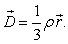(10)

Taking the divergence of both sides of equation (10), we get(11)

When all the charges in Figure 2 have a directional velocity u. The corresponding electric current density is(12)

here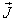is the electric current density. By substituting equation (11) and (12) into equation (8), we can also get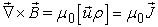(13)

Equation (12) shows that in an infinite space with uniform distribution of charges, the rotation of magnetic field generated by the charges at any point is equal to the multiplication of the electric current density and the permeability in a vacuum.

On the other hand, for the space without electric current, equation (8) can be directly transformed into(14)

Here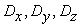stands for the electric displacement vector at x, y and z-direction respectively. From equation (14), the first term is expanded to(15)

According to the mathematics law,and, Equation (14) can be written as(16)

Because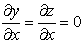, equation (16) is zero. For the y and z directions, using the same method, we obtain(17)

Substitution of equation (17) into equation (14) produces(18)

Since the velocity of the charge in y and z-directions is equal to zero, So that equation (18) can be simplified as(19)

here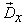is the electric displacement vector at x-direction. According to Stokes' theorem 6, we get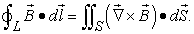(20)

Substitution equation (13) and (19) into equation (20), respectively, produces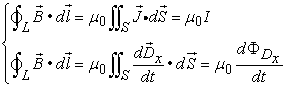(21)

hereis the electric current.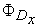is the electric displacement flux in x-direction. The first expression in equation (21) indicates that the integral of magnetic induction intensity along any closed curve is equal to the current intensity enclosed by the closed curve multiplied by the permeability in the space distributed with electric current. The second expression in equation (21) suggests that the integral of magnetic induction intensity along the closed curve in the space with no electric current is equal to rate of change of displacement flux with time in the closed curve multiplied by the permeability.

For a space, equation (21) must be expressed as(22)

And(23)

Here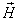is magnetic field strength. Equation(23) is completely identical with the Ampère's law in the Maxwell’s equations.

### 3. Conclusion

In this paper, we presented a new theoretical approach for a direct theory derivation of Ampère's law using the divergence and rotation of the magnetic field generated by the moving charge. This equation is exactly the same as the classical expression. This derivation in this paper can better show the physical essence of Ampere's circuital law, which also shows the transformation logic between electric and magnetic fields, which could be meaningful to young student and physical teachers.

### References

  Su zengshu. Deduction of Ampere's loop law, Journal of Guangzhou University, 1987, 01: 130-133. In article  Rajaraman K. Ampere's Magnetic Circuital Law: A Simple and Rigorous Two-Step Proof [J]. International Journal of Electrical Engineering Education, 2001, Vol. 38 Issue 3, 38-43. In article View Article  Nishmura H. From the Biot-Savart Law to Ampere's Magnetic Circuital Law via Synthetic Differential Geometry [J]. 2014, 87-89. In article  Wang Zaijun. Discussion on the application of Stokes formula and the curl of the magnetic field at the point on the infinite current carrying straight wire, College Physics, 2015(2): 20-24. In article  Zhao Haijun, Dai Jianfeng, Wang Daobin. Derivation of generalized Faraday's law and Ampere's loop law from Heisenberg equation Journal of Gansu science, 20129(4): 20-23. In article  Zhang Sanhui. College physics., electromagnetics. 1991. Volume 3 210-220. In articleThis work is licensed under a Creative Commons Attribution 4.0 International License. To view a copy of this license, visit http://creativecommons.org/licenses/by/4.0/